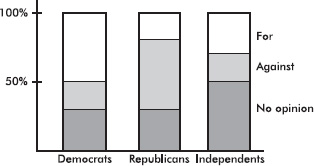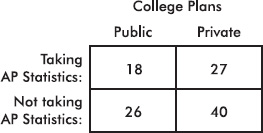# AP Statistics Multiple-Choice Practice Test 28

### Test Information10 questions23 minutes

1. PCB contamination of a river by a manufacturer is being measured by amounts of the pollutant found in fish. A company scientist claims that the fish contain only 5 parts per million, but an investigator believes the figure is higher. The investigator catches six fish that show the following amounts of PCB (in parts per million): 6.8, 5.6, 5.2, 4.7, 6.3, and 5.4. In performing a hypothesis test with H0: μ = 5 and Ha: μ > 5, what is the test statistic?

2. The distribution of weights of 16-ounce bags of a particular brand of potato chips is approximately normal with a standard deviation of 0.28 ounce. How does the weight of a bag at the 40th percentile compare with the mean weight?

3. In general, how does tripling the sample size change the confidence interval size?

4. Which of the following statements is false?

5. A study on school budget approval among people with different party affiliations resulted in the following segmented bar chart:Which of the following is greatest?

6. The sampling distribution of the sample mean is close to the normal distribution

7. What is the probability of a Type II error when a hypothesis test is being conducted at the 10% significance level (α = 0.10)?

8.Above is the dotplot for a set of numbers. One element is labeled X. Which of the following is a correct statement?

9. A 2008 survey of 500 households concluded that 82% of the population uses grocery coupons. Which of the following best describes what is meant by the poll having a margin of error of 3%?

10.The above two-way table summarizes the results of a survey of high school seniors conducted to determine if there is a relationship between whether or not a student is taking AP Statistics and whether he or she plans to attend a public or a private college after graduation. Which of the following is the most reasonable conclusion about the relationship between taking AP Statistics and the type of college a student plans to attend?